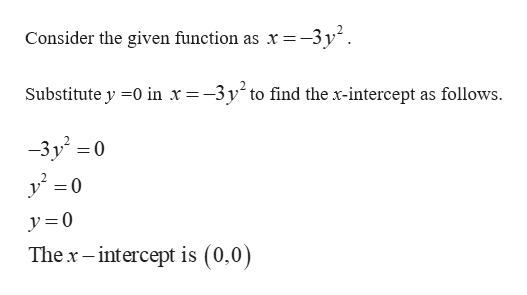# x = −3y2 Label the x- and y-intercepts. (If an answer does not exist, enter DNE.)x-intercept = y-intercept =

Question
x = −3y2

Label the x- and y-intercepts. (If an answer does not exist, enter DNE.)

x-intercept =

y-intercept =

check_circleExpert Solution
Step 1help_outlineImage TranscriptioncloseConsider the given function as x -3y*. =_ Substitute y 0 in x=-3y^ to find the x-intercept as follows -3y0 0 = y 0 The x-intercept is (0,0) fullscreen
Step 2

### Want to see the full answer?

See Solution

#### Want to see this answer and more?

Solutions are written by subject experts who are available 24/7. Questions are typically answered within 1 hour*

See Solution
*Response times may vary by subject and question
Tagged in

### Other